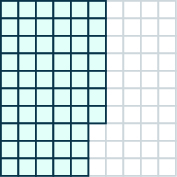## Writing Fractions and Decimals as Percents

### Learning Outcome

• Use percent to represent a given fraction or decimal

How many cents are in one dollar? There are $100$ cents in a dollar. How many years are in a century? There are $100$ years in a century. Does this give you a clue about what the word “percent” means? It is really two words, “per cent,” and means per one hundred. A percent is a ratio whose denominator is $100$. We use the percent symbol, $\%$, to show percent.

### Percent

A percent is a ratio whose denominator is $100$.

For example $46\%=\Large\frac{46}{100}$

According to data from the American Association of Community Colleges $\left(2015\right)$, about $\text{57%}$ of community college students are female. This means $57$ out of every $100$ community college students are female, as the image below shows. Out of the $100$ squares on the grid, $57$ are shaded, which we write as the ratio $\Large\frac{57}{100}$.

Among every $100$ community college students, $57$ are female.Similarly, $\text{25%}$ means a ratio of $\Large\frac{25}{100}\normalsize ,\text{3%}$ means a ratio of $\Large\frac{3}{100}$ and $\text{100%}$ means a ratio of $\Large\frac{100}{100}$. In words, “one hundred percent” means the total $\text{100%}$ is $\Large\frac{100}{100}$, and since $\Large\frac{100}{100}\normalsize =1$, we see that $\text{100%}$ means $1$ whole.

To convert a decimal to a percent, remember that percent means per hundred. If we change the decimal to a fraction whose denominator is $100$, it is easy to change that fraction to a percent.

### Convert a decimal to a percent

1. Write the decimal as a fraction.
2. If the denominator of the fraction is not $100$, rewrite it as an equivalent fraction with denominator $100$.
3. Write this ratio as a percent.

### example

Convert each decimal to a percent:

1. $0.05$

2.$0.83$

Solution

 1. $0.05$ Write as a fraction. Five hundredths – the denominator is $100$. ${\Large\frac{5}{100}}$ Write this ratio as a percent. $5$%
 2. $0.83$ Write as a fraction. Eighty-three hundredths – the denominator is $100$. ${\Large\frac{83}{100}}$ Write this ratio as a percent. $83$%

### try it

Let’s look at a few more examples of converting decimals to percents, but these aren’t as straight forward!

### example

Convert each decimal to a percent:
1. $0.2$

2. $1.05$

2. $0.075$

### try it

Let’s summarize some of the results from the previous examples in the table below so we can look for a pattern.

Decimal Percent
$0.05$ $\text{5%}$
$0.83$ $\text{83%}$
$0.2$ $\text{20%}$
$1.05$ $\text{105%}$
$0.075$ $\text{7.5%}$

Do you see the pattern? To convert a decimal to a percent, we move the decimal point two places to the right and then add the percent sign.

The next table uses the decimal numbers in the table above and shows visually to convert them to percents by moving the decimal point two places to the right and then writing the $\%$ sign.

Percent Decimal
$\text{6%}$ $0.06$
$\text{78%}$ $0.78$
$\text{135%}$ $1.35$
$\text{12.5%}$ $0.125$

In an earlier lesson, we learned how to convert fractions to decimals. Now we also know how to change decimals to percents. So to convert a fraction to a percent, we first change it to a decimal and then convert that decimal to a percent.

### Convert a fraction to a percent

1. If possible, convert the fraction to a denominator of $100$.
2. If not, convert the fraction to a decimal by dividing.
3. Convert the decimal to a percent.

### example

Convert each fraction or mixed number to a percent:

1.  ${\Large\frac{3}{4}}$

2.  ${\Large\frac{11}{8}}$

3.  $2{\Large\frac{1}{5}}$

Sometimes when changing a fraction to a decimal, the division continues for many decimal places and we will need to round off the quotient. Typically, you will round before converting to a percent unless instructed otherwise. The number of decimal places we round to will depend on the situation. If the decimal calculation involves money, we round it to the hundredths place. For most other cases, we will round the number to the nearest thousandth, so the percent will be rounded to the nearest tenth.

## Contribute!

Did you have an idea for improving this content? We’d love your input.